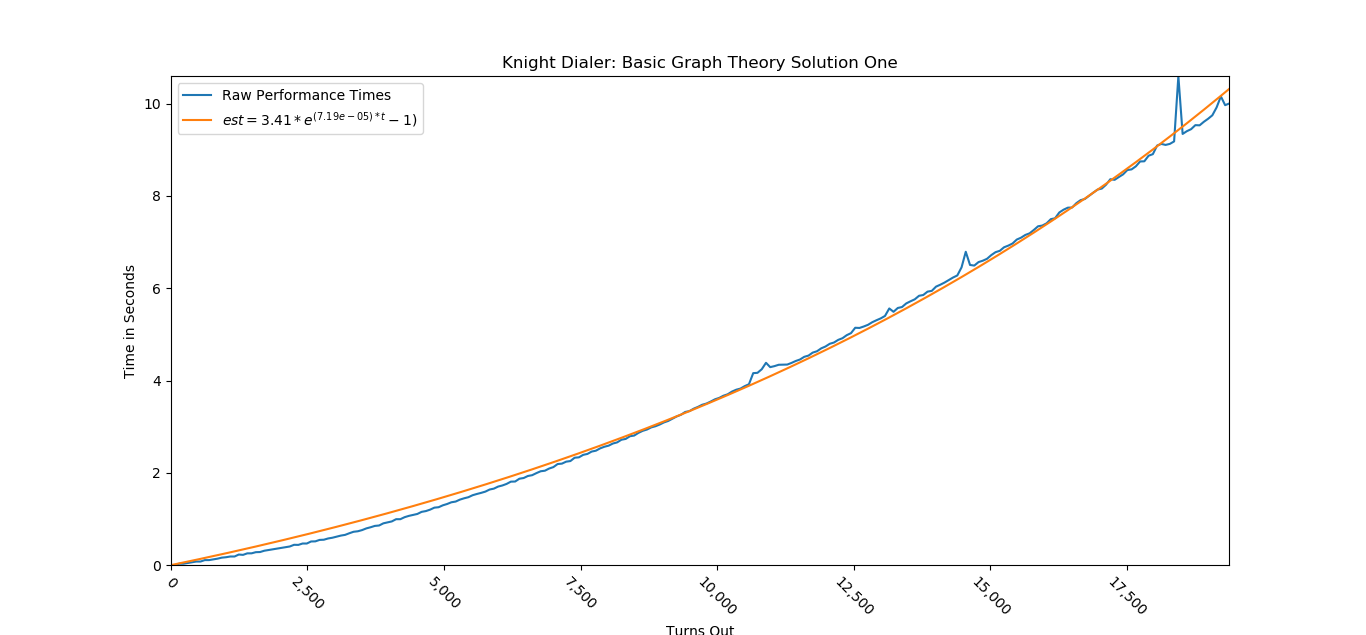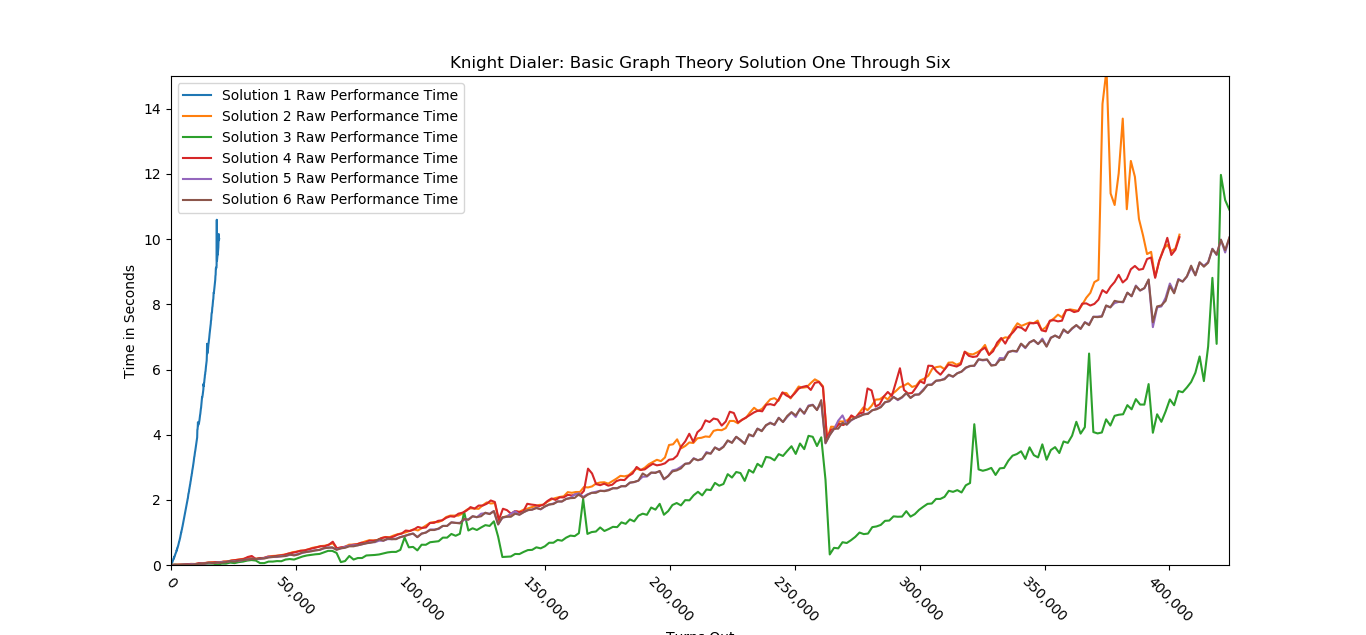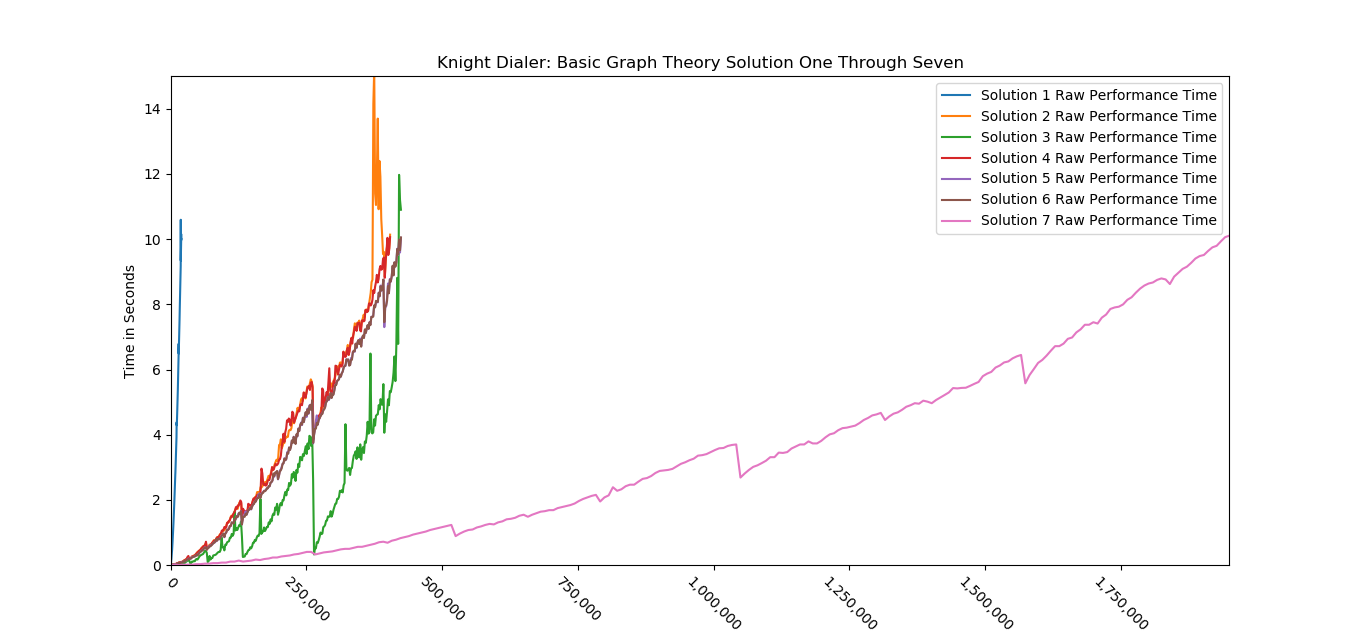# Solving Google’s Knight Dialer With Graph Theory: Part 2“Knight to E4!…. Yes Hermione, I think this is going to be exactly like wizard’s chess.” — Ronald Weasley

# Binary Decomposition

In Solving Google’s Knight Dialer With Graph Theory: Part 1, we were essentially taking 1 step forward at a time until we reach the matrix we need. However, we don’t have to take 1 step at a time; we can take many steps forward with a technique called Binary Decomposition.

The simplest introduction to Binary Decomposition is often the example of multiplication.

Let’s say we have to solve x=3⁸. We could multiply “3” 8 times and we would get our answer. But we know that 3² =9 and we could use substitution and only need to solve x=9⁴. But we also know 9² =81, we could substitute that and only have to find x=81² which equals 6561.

81² might be difficult to do for you and me, but its less work for a computer than 3⁸ as there are fewer operations. With Binary Decomposition, instead of 7 separate multiplications, a computer would only need 3. This allows the work to grow logarithmically instead of linearly as we need to multiply larger and larger powers.

But how would we solve for 3¹⁰? 10 isn’t a power of 2. Well, all integers can be expressed as a series of powers of 2. 10= 2¹ *+ 2³ because 2¹ is 2 and 2³ is 8, 2+ 8=10. So, x =3¹⁰ = 3²*3⁸= 3²*(3²)⁴ = 9*9⁴= 9*(9²)² = 9*81² = 9*6561 = 59049. Again, this is a lot less work for a computer.

This can all be applied to square matrices, too.

# Binary Decomposition Applied

One way to implement Binary Decomposition is with recursion. There are three situations we need to be able to handle.

1. Our exit condition, which will be when the number of turns is equal to one
2. Our condition when the number of turns is equal to 2 to some power
3. Our condition when the number of turns is not equal to 2 to some power

Now, this program could be calculated entirely with recursion and it would be effective. At 5000 turns, our final linear solution from the previous article took 1.387 seconds. A recursive solution without memoization took about .02 seconds. With memoization, it took about .014 seconds.

However, as stated in Alex’s article, recursion has some problems. It grows in memory requirements as the inputs get larger and is limited to 1000 recursive calls on Linux & Mac OS and limited to 2000 calls on Windows OS.

However, I hate recursion. It is a pain to test and maintain. I don’t like how easy it is to create an infinite loop and it feels like a step backwards to use it.

So, let’s get rid of it.

# Non-Recursive Knight Solver

In our recursive solution, we solved the problem using Binary Decomposition and we are going to do the same thing here. There are two recursive paths that need to be handled: breaking the problem into powers of 2 and calculating the matrix raised to a power of 2

The first part is responsible for calculating M^N where N= 2^n. It can be phased out by replacing it with a simple function that takes a starting matrix & “n”. Then, the function squares the matrix “n” times and returns it.

So, for 8 turns out, we would pass 3 as the baseTwoTurns because 2 raised to the 3rd power is 8. In this function, the first pass would generate M², the second pass would generate M⁴, and the third and final pass would generate M⁸.

We can implement this function into our Fully Recursive solution with only a slight modification.

The second recursive path is responsible for breaking the matrix calculation for N turns into a series of base two powers. So 11 breaks into [8, 2, 1] which can be expressed as [2³, 2¹, 2⁰]; So, the array we need [3, 1, 0].

We can calculate this by iteratively removing the greatest power of two from our original number until it is equal to zero.

You may have noticed that with our current getBaseTwoTurnsMatrix function, we will not be able to take advantage of cacheing. In our recursive solution, calling getMatrix for 8 turns out will eventually call getMatrix for 2 turns out. That is not true for this implementation.

We can circumvent this limitation by going from smallest square to largest square, storing the result from the last turn, and only squaring that matrix by the difference between the two powers.

For example, if we were going 24 turns out, 24 breaks down to [2³ ,2⁴]. We take M¹ and square it 3 times to get M⁸. By saving M⁸, we only have to square the M⁸ matrix once to get to M¹⁶. Then, we only have to multiply M⁸ & M¹⁶ to get M²⁴.

# Out of the Box

Trying to come up with everything we have done, in 45 minutes, would be a lot of work; Fortunately, you don’t have to. Everything in getMatrix has already been implemented in Numpy’s Linalg.matrix_power function and can be applied to any square matrix.

Unfortunately, we are now butting up against the limits of the implementation of the matrix multiplication in NumPy. In fact, due to the sheer size of the numbers we are working with all of our implementations grow exponentially as the number of turns out increase. The difference being how quickly they grow exponentially. There isn’t anything we could do to improve the matrix multiplication, but we could use a better matrix.

# Same Answer, Different Graph

Lets take a look at our original Neighbor Diagram:

In the Neighbor diagram, a lot of the numbers look very similar. “4” & “6” are always connected to “0” and two “corner” positions (1,3,7,9). Lets “4” & “6” call a “bridge” position. “2” & “8” are very similar, they always connect to two corner positions. Lets call them an “edge” position. And lets call “0” a center position. we can handle “5” as an edge case and use the following mapping:

A. Corners: (1, 3, 7, 9)
B. Edges: (2, 8)
C. Bridges: (4,6)
D. Center: (0)

By relabeling the first state diagram with the letters above, we can see the similarity:

“A” (or a Corner) always leads to 1 “B” (or Edge) and 1 “C” (or a Bridge). “B” always leads to 2 “A”s. “C” always leads to 2 “A”s and 1 “D” (or center). “D” always leads to 2 “C”s.

A: 1-B, 1-C
B: 2-A
C: 2-A, 1-D
D: 2-C

Since all the corners behave the same, it doesn’t matter which corner the knight is at; the number of next numbers that can be dialed is the same. The same is true for edges or for bridges.

We are exploiting the graph’s symmetry to generate a new simpler graph that is still capable of answering our problem. This new graph may not be able to answer all the questions our original graph could, but it can still answer our problem.

Since we have a mapping from one state ( “A”, “B”, “C”, “D”) to another, it can be drawn as a new state diagram with a corresponding transition matrix.

Why is this a better map to use? Well, the number of multiplication operations to multiply two NxN matrices is equal to the N³.

So, our original 10x10 matrix requires 1000 multiplication operations each time we multiplied it by itself, while our new 4x4 matrix only requires 64 multiplication operations.

If we needed to calculate M⁸ we would need to square our matrix 3 times. For our original matrix, that would be 3000 multiplication operations. With our New matrix, it would only require 192 multiplication operations, only 6.4% of the operations the original matrix required. This represents a significant reduction in the cost to calculate each matrix.

However, this new matrix requires another layer of abstraction to map our the starting position on our original matrix to the positions on our new matrix.

Implementing only requires a slight modification of our function.

# How Much Better Is It?

Well, here is a visualization of the performance for our Linear solution:All data was generated by measuring the time to complete with a starting position of 0

In this visualization, I measured the time to complete for 250 different turns equally spaced from 0 to 20,000. There is a bit of noise in the data, this may have been due to:

• me, idly hitting the space bar to see if it was still working
• a random background update from windows
• magic smoke in computer decided to be slow

Here is a visualization of the raw performance data for the solutions we’ve done so far.Solution 1 = linear solution, Solution 2 = Recursive solution, Solution 3 = Recursive solution + Memoization, Solution 4 = dynamic programing solution without reversing the powers, Solution 5 = dynamic programming solution with reversing the powers, Solution 6 = Nump’s matrix power solution

There are several interesting things to point out in this graph.

1. Every implementation of Binary Decomposition is better than our first solution.
Hooray!
2. There is some noise, but there are also some patterns for solutions two through six.
The sudden drops in run time are due to how binary decomposition works. In general, it easier to calculate 2^N turns out than (2^N)-1. In Binary decomposition, we have to calculate each power of 2, but we then have to combine each of the factors. So, there will be an increasingly large drop in runtime upon reaching a power of 2.
3. Solution 3, the recursive solution + memoization, performs really well
While benchmarking, the cache was cleared between each run as the point was to was to measure individual performance at a number of turns.
It still does really well, but the problems start to show towards the tail end of the graph. If we were to use this solution only once for 400,000 turns out, getMatrix would be storing nineteen 10x10 matrices with huge numbers in memory. Its a significant cost to memory that will significantly degrade performance. In fact, Its pretty easy to see the performance start to degrade significantly towards the end of the graph.
4. Solutions 5 & 6 are identical in performance.
Solution 5 & 6 are the same algorithm; So, their performances will be the same. However, NumPy’s matrix_power is less verbose than Solution 5’s getMatrix and has more error handling around inputs and handles negative exponents. I’d recommend using it.

Lets see how our final solution compares.

# Lucky Number 7= condensed graph solutionFun fact: at 1,750,000 turns, the number would be somewhere between 10⁵²⁶⁸⁰² and 10⁸³⁴⁹⁶³, an absolutely incomprehensibly large number

The final solution blows the competition out of the water; It is consistently faster than the previous six solutions even for lower number of turns. There is significantly less information to store as there are fewer positions and fewer relationships. This flattens the performance curve, limiting the impact of memory issues resulting from the increasingly large numbers

# Wait, weren’t these O(log(N))?

You may be wondering why these curves don’t seem to be logarithmic. Well, we’re actually running into the performance curve of multiplication. Since, the numbers are beyond astronomically large, multiplication is starting to take up the most amount of time.

# How much further we can go?

We could look into how the multiplication algorithm is implemented and implement a faster algorithm like the FASTEST multiplication algorithm ever from David Harvey and Joris van der Hoeven

If this was implemented into a service, there may be ways to do distributed computing or store commonly calculated matrices. We could also leverage a GPU to do the calculations. We could also switch to a language other than python for something closer to bare metal performance.

This is an educated guesstimate. Running the calculations on a GPU might do a 10² improvement depending on the parallelization. We could get a 10³ improvement by throwing it at a sufficiently large computing cluster which really isn’t crazy. A C implementation could maybe get a 10² to 10³ improvement from what little research I have done. I’m not sure how much the new multiplication algorithm would do. We could also precalculate and store some of the common multiples of 2-power matrices.

An ML engineer would know better, but I think all these ideas together could feasibly improve the performance from ~10⁶ steps in <10 seconds to >10¹³ of steps in <10 seconds. Assuming, we don’t run into a different constraint at that point like Skynet deciding this is robot cruelty.

However, we are at a pretty good stopping point. We have hit the limits of graph theory. If anyone figures out how to implement Dr. Harvey and Dr. van der Hoeven’s multiplication or any of the other improvements, please let me know! I’d love to see it.

--

--